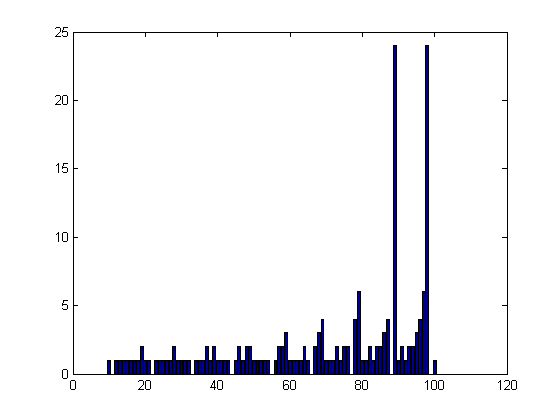## Loren on the Art of MATLABTurn ideas into MATLAB

Note

Loren on the Art of MATLAB has been archived and will not be updated.

# Palindrome Numbers

Today I'd like to introduce a guest blogger, Mike Garrity, who is a graphics software developer at The MathWorks. Although his degree was in computational fluid dynamics, he has spent most of the last 25 years designing graphics hardware, rendering pipelines, and visualization software.

### Introduction

Some numbers like 323 are palindromes. Other numbers like 124 are not. But look what happens when I add that number to a reversed copy of itself.

 124
+421
----
545

Let’s try another.

 150
+051
----
201

No, that didn’t work, but what if I keep going?

 201
+102
----
303

There, it became a palindrome again. Let’s try another one.

 291
+192
----
483
 483
+384
----
867
 867
+768
----
1635
 1635
+5361
-----
6996

That took several turns, but it did become a palindrome. I wonder what the pattern is. Let’s write a program to find out. I'll have it return the number of iterations and the final number.

### The Code

Here's the code for producing palindrome numbers.

type pal196
function [n p] = pal196(in)
% Compute the number of iterations of
% reverse and add which are required
% to convert a number into a palindrome.
%
% Here are some fun ones.
% - 147996
% - 1000689
% - 9008299
%

n = 0;
maxn = 10000;
a = num_to_array(fix(in));
while ~all(a == fliplr(a))
n = n+1;

if (n > maxn)
error('Palindrome:TooManyDigits', ...
['Giving up on ', num2str(in), ' at ' num2str(n), ...
' iterations, value = ' array_to_str(a)]);
end
end

if (nargout == 2)
p = array_to_str(a);
end
end

function a = num_to_array(in)
% Convert a number into an array of digits
%
a = [];
while (in >= 1)
a = [mod(in, 10), a];
in = floor(in/10);
end
end

% Add an array of digits to a reversed copy
% of itself.
%
out = in + fliplr(in);
for idx=size(out,2):-1:1
carry = 0;
if (out(idx) >= 10)
carry = 1;
out(idx) = mod(out(idx),10);
end
if (carry > 0)
if (idx > 1)
out(idx-1) = out(idx-1) + carry;
else
out = [carry out];
end
end
end
end

function str = array_to_str(a)
% Format an array of digits as a text string.
%
len = length(a);
if len > 3
end
end



### Explore the First Few Numbers

If I run that for all of the numbers from 1 to 100 and plot the number of iterations, I get this plot.

counts = zeros(1,100);
for idx=1:100
counts(idx) = pal196(idx);
end
bar(counts);That’s interesting. It looks like there’s a pattern, but I don’t quite get it. Let's keep going.

counts=zeros(1,200);
firstfailed = [];
try
for idx=1:200
counts(idx) = pal196(idx);
end
catch ME
firstfailed = idx;
end
if ~isempty(firstfailed)
disp(['Failure occurred for n = ', num2str(idx)])
end
if exist('ME', 'var')
ME
ME.message(1:45)
[ME.message(46:72),'..........', ME.message(end-27:end)]
end
Failure occurred for n = 196
ME =
MException

Properties:
identifier: 'Palindrome:TooManyDigits'
message: [1x5591 char]
cause: {}
stack: [6x1 struct]
ans =
Giving up on 196 at 10001 iterations, value =
ans =
6,643,864,828,137,164,591,..........,691,854,727,307,295,584,356


For the number 196, it quit at 10,000 iterations. At that point, the number had 4,159 digits. Wow, that's a big number!

### Does It Ever Become a Palindrome?

So 196 doesn't work. Or does it? What if we ran it longer before giving up? Do you think that it would converge on a palindrome if we went further?

How far can you run it out?

Before we try to go too far, perhaps we should optimize the code a bit. What could you change to make it run faster and use less memory?

### References

Here's a few interesting references to follow up on this topic.

### Other Values?

What other numbers can you find that behave like 196? Also, some of the numbers that don't seem to converge seem to fall into a small number of common patterns. Can you identify any of these (by numeric experimentation preferably, not web look up)? Please add your observations here.

Published with MATLAB® 7.8